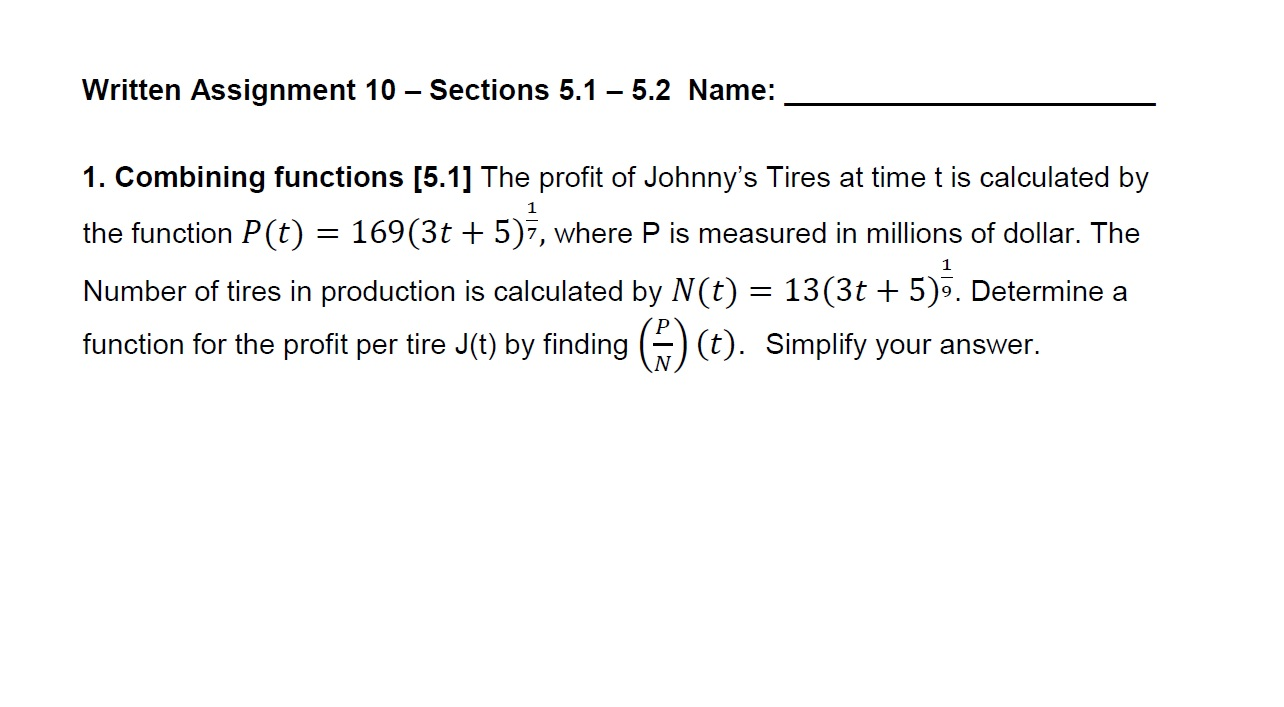# Written Assignment 10 - Sections 5.1 - 5.2 Name:1. Combining functions [5.1] The profit of Johnny's Tires at time t is calculated bythe function P(t) 169(3t + 5)7, where P is measured in millions of dollar. TheNumber of tires in production is calculated by N(t) 13(3t 5). Determine afunction for the profit per tire J(t) by fnding )(t) Simplify your answer.11

Question
59 viewshelp_outlineImage TranscriptioncloseWritten Assignment 10 - Sections 5.1 - 5.2 Name: 1. Combining functions [5.1] The profit of Johnny's Tires at time t is calculated by the function P(t) 169(3t + 5)7, where P is measured in millions of dollar. The Number of tires in production is calculated by N(t) 13(3t 5). Determine a function for the profit per tire J(t) by fnding )(t) Simplify your answer. 1 1 fullscreen
check_circle

Step 1

To simplify the expression for the quotiient J(t)=P(t)/N(t)

Step 2

### Want to see the full answer?

See Solution

#### Want to see this answer and more?

Solutions are written by subject experts who are available 24/7. Questions are typically answered within 1 hour.*

See Solution
*Response times may vary by subject and question.
Tagged in

### Exponents